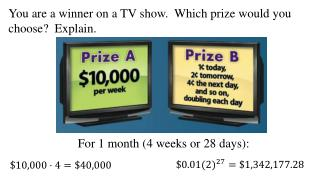Download PresentationYou are a winner on a TV show. Which prize would you choose? Explain.# You are a winner on a TV show. Which prize would you choose? Explain. - PowerPoint PPT Presentation

Download Presentation##### You are a winner on a TV show. Which prize would you choose? Explain.

Download Policy: Content on the Website is provided to you AS IS for your information and personal use and may not be sold / licensed / shared on other websites without getting consent from its author. While downloading, if for some reason you are not able to download a presentation, the publisher may have deleted the file from their server.

- - - - - - - - - - - - - - - - - - - - - - - - - - - E N D - - - - - - - - - - - - - - - - - - - - - - - - - - -
##### Presentation Transcript

1. You are a winner on a TV show. Which prize would you choose? Explain. For 1 month (4 weeks or 28 days):

2. 7-5Exponential & Logarithmic Equations Today’s Objective: I can solve exponential & logarithmic equations. We will round all answer to the ten-thousandth.

3. Exponential Equation: Common Base Write each side of equation with same base number. Set exponents equal to each other and solve.

4. Exponential Equation – Different Bases Isolate exponential term. Take the logarithm of both sides. Solve. Isolate exponential term. Convert to logarithm form Solve.

5. Logarithmic Equation Isolate logarithm to a single term. Convert to exponential form Solve Pg. 473 # 7-21 odds, 31-47 odds